Courses

# Response Of Single Degree Of Freedom Systems To Nonperiodic Inputs Mechanical Engineering Notes | EduRev

## Mechanical Engineering : Response Of Single Degree Of Freedom Systems To Nonperiodic Inputs Mechanical Engineering Notes | EduRev

The document Response Of Single Degree Of Freedom Systems To Nonperiodic Inputs Mechanical Engineering Notes | EduRev is a part of the Mechanical Engineering Course Mechanical Vibrations.
All you need of Mechanical Engineering at this link: Mechanical Engineering

Physics of Impulsive Motion

Recall from dynamics that the principle of impulse and momentum for a particle states that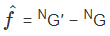(2–1)

where NG  is the linear momentum of the particle as viewed by an observer in an inertial reference frame N. Suppose now that we consider the following system. A block of mass m is connected to a linear spring with spring constant K and unstretched length ℓ0 and a viscous linear damper with damping coefficient c as shown in Fig. 2–1. The block is initially at rest (i.e., its initial velocity is zero) at its static equilibrium position (i.e., the spring is initially unstressed) when a horizontal impulse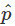is applied. We are interested here in determining the velocity of the block immediately after the application of the impulse.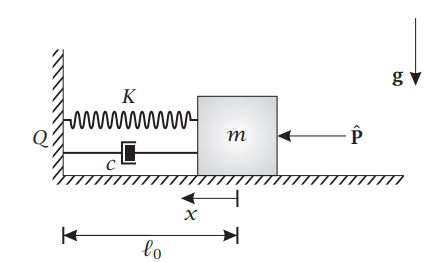Figure 2–1 Block of mass m connected to linear spring and linear damper struck by horizontal impulse.

The solution of the above problem is found as follows. First, let F be the ground. Then,

choose the following coordinate system fixed in F:

Origin at block
when x = 0

Ex = To the left
Ez = Into page
Ey = Ez × Ex

Then, the position of the block is given in terms of the displacement x as

r = xEx                                                                                                                     (2–2)

Because {Ex, Ey , Ez} is a fixed basis, the velocity of the block in reference frame F is given as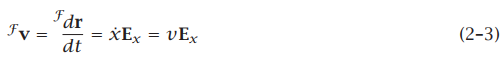Now because we are going to apply the principle of linear impulse and momentum to this problem, we do not need the acceleration of the block. Instead, we know that neither the spring nor the damper can apply an instantaneous impulse. Therefore, the only impulse applied to the system at t = 0 is that due to. Consequently, the external impulse acting on the system at t = 0 is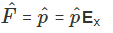(2–4)

Furthermore, the linear momentum of the block the instant before the impulse is applied is zero (i.e., the block is initially at rest) while the linear momentum of the block the instant after the impulse is applied is given as

FG′ = m F v ′ = mv′Ex                                                                                              (2–5)

Setting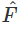equal to FG′ , we obtain= mv′ ≡ mv(t = 0+)                                                                                               (2–6)

Solving for v(t =0+), we obtain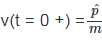(2–7)

The result of this analysis shows that the response of a resting second-order linear system to an impulsive forceis equivalent to giving the system the initial velocity shown in Eq. (2–7).

Offer running on EduRev: Apply code STAYHOME200 to get INR 200 off on our premium plan EduRev Infinity!

30 docs

,

,

,

,

,

,

,

,

,

,

,

,

,

,

,

,

,

,

,

,

,

;